# SRCMS 多处越权+权限提升管理员漏洞

SRCMS是一个开源的企业安全应急响应中心，基于ThinkPHP框架开发。我将ThinkPHP归为半现代框架，因为它在近些年的发展中已经越来越多地引入现代化的一些元素（命名空间、ORM等）。当然也由于这些元素的引入，将会造成一些以前写代码不会遇到的安全问题。

## 0x01 ThinkPHP特性造成的越权漏洞

/Application/User/Controller/PostController.class.php:77

```<?php
/**
*查看漏洞报告
*/
public function view(){
\$id = session('userId');
\$rid = I('get.rid',0,'intval');
\$model = M("Post");
\$post = \$model->where('user_id='.\$id)->where('id='.\$rid)->find();
\$tmodel= M('setting');
\$title = \$tmodel->where('id=1')->select();
\$this->assign('title', \$title);
\$this->assign('model', \$post);
\$this->display();
}
```

view函数存在一个越权查看他人漏洞报告的漏洞，为什么？他这里明明有`where('user_id='.\$id)`，查询了user_id的呀？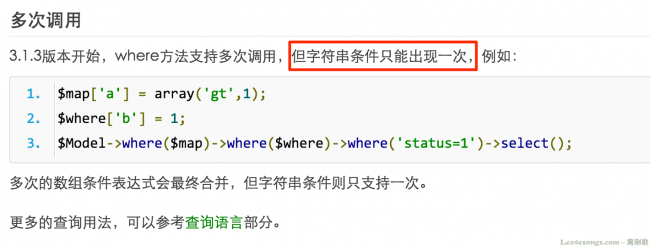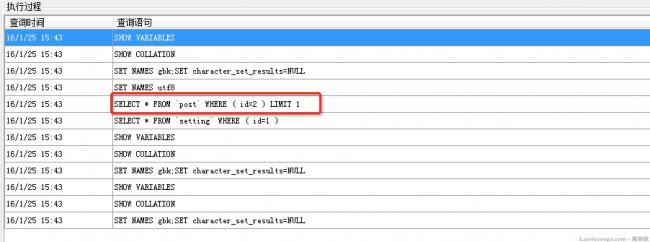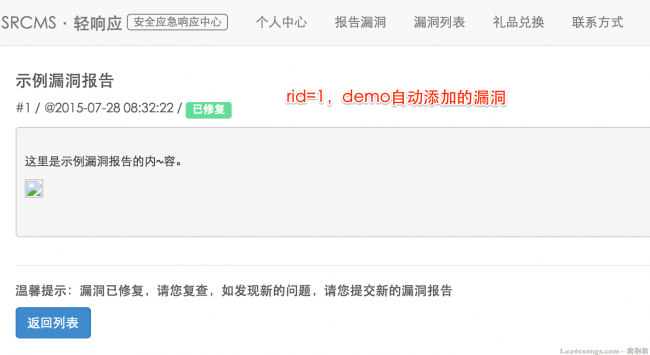## 0x02 Model误用造成的越权漏洞

/Application/User/Controller/InfoController.class.php:56

```<?php
public function update()
{
//默认显示添加表单
\$id = session('userId');
if (!IS_POST) {
\$info = M('info')->where('user_id='.\$id)->select();
\$this->assign('info',\$info);
\$this->display();
}
if (IS_POST) {
//如果用户提交数据
\$model = D("info");
\$model->user_id = 1;
if (!\$model->create()) {
// 如果创建失败 表示验证没有通过 输出错误提示信息
\$this->error(\$model->getError());
exit();
} else {
if (\$model->save()) {
\$this->success("更新成功", U('info/index'));
} else {
\$this->error("更新失败");
}
}
}
}
```

```<?php
public function create(\$data='',\$type='') {
// 如果没有传值默认取POST数据
if(empty(\$data)) {
\$data   =   I('post.');
}elseif(is_object(\$data)){
\$data   =   get_object_vars(\$data);
}
// 验证数据
if(empty(\$data) || !is_array(\$data)) {
\$this->error = L('_DATA_TYPE_INVALID_');
return false;
}

// 状态
\$type = \$type?:(!empty(\$data[\$this->getPk()])?self::MODEL_UPDATE:self::MODEL_INSERT);

...

// 创建完成对数据进行自动处理
\$this->autoOperation(\$data,\$type);
// 赋值当前数据对象
\$this->data =   \$data;
// 返回创建的数据以供其他调用
return \$data;
}
```

```<?php
private function autoOperation(&\$data,\$type) {
if(!empty(\$this->options['auto'])) {
\$_auto   =   \$this->options['auto'];
unset(\$this->options['auto']);
}elseif(!empty(\$this->_auto)){
\$_auto   =   \$this->_auto;
}
// 自动填充
if(isset(\$_auto)) {
foreach (\$_auto as \$auto){
// 填充因子定义格式
// array('field','填充内容','填充条件','附加规则',[额外参数])
if(empty(\$auto)) \$auto =  self::MODEL_INSERT; // 默认为新增的时候自动填充
if( \$type == \$auto || \$auto == self::MODEL_BOTH) {
if(empty(\$auto)) \$auto =  'string';
switch(trim(\$auto)) {
case 'function':    //  使用函数进行填充 字段的值作为参数
case 'callback': // 使用回调方法
\$args = isset(\$auto)?(array)\$auto:array();
if(isset(\$data[\$auto])) {
array_unshift(\$args,\$data[\$auto]);
}
if('function'==\$auto) {
\$data[\$auto]  = call_user_func_array(\$auto, \$args);
}else{
\$data[\$auto]  =  call_user_func_array(array(&\$this,\$auto), \$args);
}
break;
case 'field':    // 用其它字段的值进行填充
\$data[\$auto] = \$data[\$auto];
break;
case 'ignore': // 为空忽略
if(\$auto===\$data[\$auto])
unset(\$data[\$auto]);
break;
case 'string':
default: // 默认作为字符串填充
\$data[\$auto] = \$auto;
}
if(isset(\$data[\$auto]) && false === \$data[\$auto] )   unset(\$data[\$auto]);
}
}
}
return \$data;
}
```

```<?php
namespace User\Model;
use Think\Model;
class InfoModel extends Model{

protected \$_validate = array(
array('realname','require','请填写真实姓名'), //默认情况下用正则进行验证
array('zipcode','require','请填写邮编'), //默认情况下用正则进行验证
array('location','require','请填写地址'), //默认情况下用正则进行验证
array('tel','require','请填写联系电话'), //默认情况下用正则进行验证
array('alipay','require','请填写支付宝账号，方便发放现金奖励'), //默认情况下用正则进行验证
);

protected \$_auto = array (
array('user_id','getUid',1,'callback'), // 对update_time字段在更新的时候写入当前用户ID
);

protected function getUid(){
return session('userId');
}

}
}
```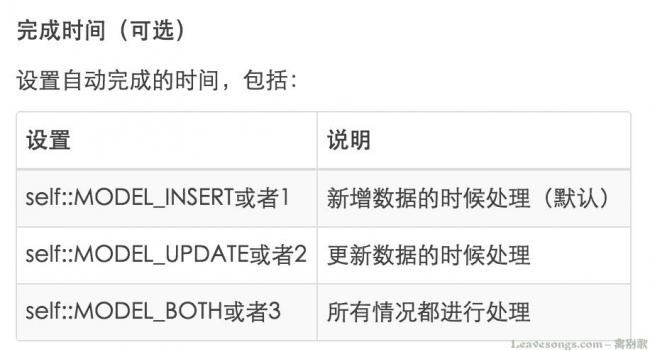1代表的是insert，只有在insert的时候才进行处理。

```<?php
// 状态
\$type = \$type?:(!empty(\$data[\$this->getPk()])?self::MODEL_UPDATE:self::MODEL_INSERT);
```

```<?php
//type是update（2），但\$auto却是 1, \$type==\$auto不成立
if( \$type == \$auto || \$auto == self::MODEL_BOTH) {
```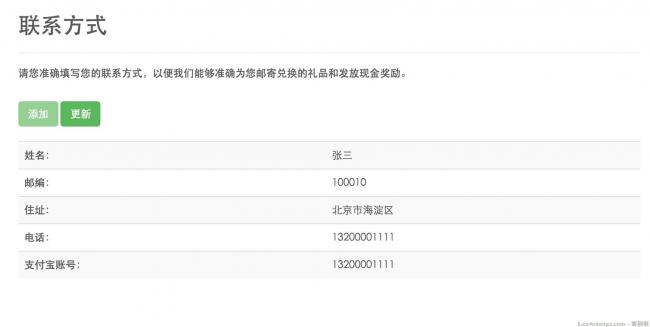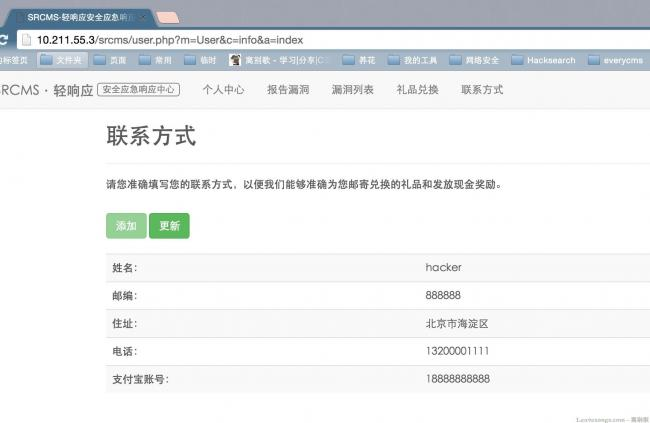## 0x03 横向挖掘 —— 权限提升漏洞（可获取管理员权限）

```<?php
{
//默认显示添加表单
if (!IS_POST) {
\$this->display();
}
if (IS_POST) {
//如果用户提交数据
\$model = D("Member");
if (!\$model->create()) {
// 如果创建失败 表示验证没有通过 输出错误提示信息
\$this->error(\$model->getError());
exit();
} else {
\$this->success("用户添加成功", U('index/index'));
} else {
\$this->error("用户添加失败");
}
}
}
}
```

```<?php
namespace User\Model;
use Think\Model;
class MemberModel extends Model{
protected \$_validate = array(
array('email','require','请填写邮箱！'), //默认情况下用正则进行验证
array('email','email','邮箱格式错误！'), //默认情况下用正则进行验证
array('email','','邮箱已存在！',0,'unique',self::MODEL_BOTH), // 在新增的时候验证name字段是否唯一
);

protected \$_auto = array(
array('update_at','time',2,'function'), //更新时
array('create_at','time',1,'function'), //新增时
);
}
```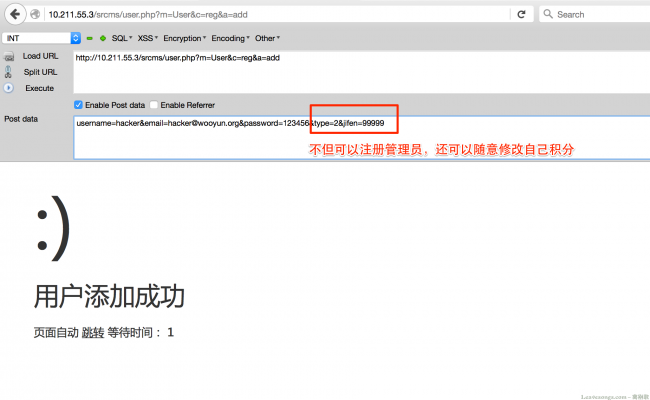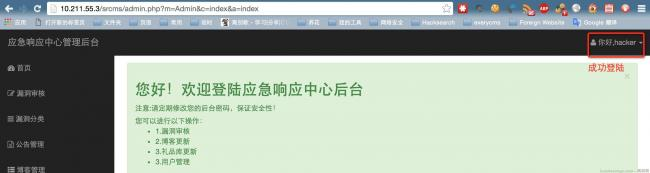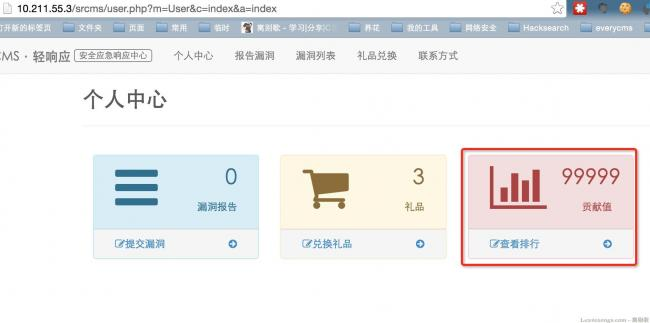# 赞赏# 评论hdphp 回复